# Solved 2018 Question Paper ICSE Class 10 Physics

## Section I (40 Marks)

#### Question 1(a)

(i) State and define the S.I. unit of power. 

(ii) How is the unit horse power related to the S.I. unit of power ?

(i) The S.I. unit of power is watt

It is defined as the power spent when 1 joule of work is done for 1 second.

(ii) 1 horse power = 746 watt.

#### Question 1(b)

State the energy changes in the following cases while in use: 

(i) An electric iron.

(ii) A ceiling fan.

(i) In an electric iron, the electrical energy changes into heat energy.

(ii) In a ceiling fan, the electrical energy changes into mechanical energy.

#### Question 1(c)

The diagram below shows a lever in use: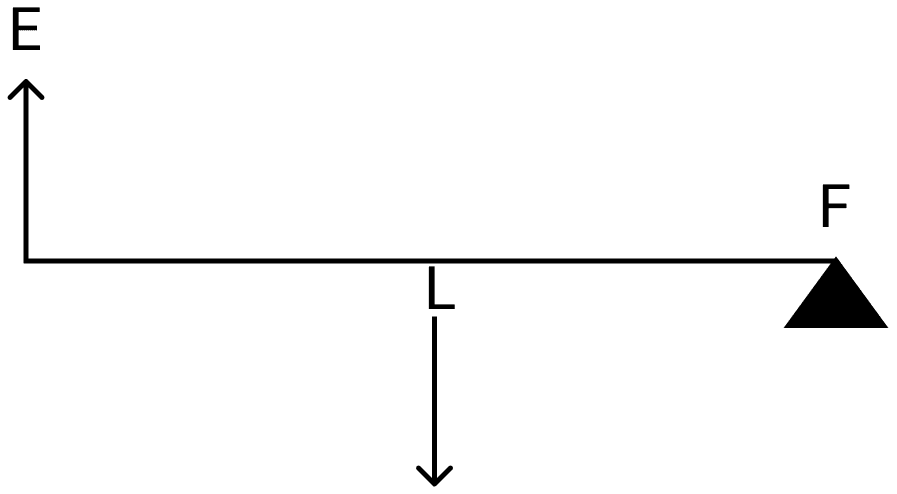(i) To which class of levers does it belong?

(ii) Without changing the dimensions of the lever, if the load is shifted towards the fulcrum what happens to the mechanical advantage of the lever?

(i) It belongs to second class lever.

(ii) The mechanical advantage of the lever will increase when load is shifted towards the fulcrum.

#### Question 1(d)

(i) Why is the ratio of the velocities of light of wavelengths 4000 Å and 8000 Å in vacuum 1 : 1 ?

(ii) Which of the above wavelengths has a higher frequency? 

(i) In vacuum the light of each wavelength travels with the same speed i.e., the speed of light of wavelength of 4000 Å is same as that of light of wavelength 8000 Å.

(ii) The light of wavelength 4000 Å will have higher frequency.

#### Question 1(e)

(i) Why is the motion of a body moving with a constant speed around a circular path said to be accelerated? 

(ii) Name the unit of physical quantity obtained by the formula $\dfrac{2K}{V^2}$.
Where K: kinetic energy, V: Linear Velocity.

(i) The motion of a body moving with a constant speed around a circular path is said to be accelerated because as the direction keeps changing the velocity changes and therefore it's an accelerated motion.

(ii) $\dfrac{2K}{V^2}$ = $\dfrac{2 \times (\dfrac{1}{2} \times mV^2)}{V^2}$ = m.

Therefore, the quantity, $\dfrac{2K}{V^2}$ will have the unit of mass m which is kilogram (kg).

#### Question 2(a)

The power of a lens is –5D. 

(i) Find it's focal length.

(ii) Name the type of lens.

(i) Given, P = -5D

Focal length f = $\dfrac{1}{P}$ = $\dfrac{1}{-5}$ = -0.2 m = -20 cm

(ii) The lens is concave.

#### Question 2(b)

State the position of the object in front of a converging lens if: 

(i) It produces a real and same size image of the object.

(ii) It is used as a magnifying lens.

(i) The object is placed at a distance of 2f in front of the lens.

(ii) The object is placed between optical centre and focus of the lens.

#### Question 2(c)

(i) State the relation between the critical angle and the absolute refractive index of a medium.

(ii) Which colour of light has a higher critical angle? Red light or Green light. 

(i) μ = $\dfrac{1}{\text{sin C}}$ where μ is the refractive index of the medium and C is the critical angle.

(ii) The critical angle of Red light is higher than that of green light.

#### Question 2(d)

(i) Define scattering. 

(ii) The smoke from a fire looks white.

Which of the following statements is true?

1. Molecules of the smoke are bigger than the wavelength of light.
2. Molecules of the smoke are smaller than the wavelength of light.

(i) Scattering of light is the process of absorption and remission of light energy by the dust particles and air molecules present in the atmosphere.

(ii) Statement 1 is correct, i.e., molecules of the smoke are bigger than the wavelength of light.

#### Question 2(e)

The following diagram shows a 60°, 30°, 90° glass prism of critical angle 42°. Copy the diagram and complete the path of incident ray AB emerging out of the prism marking the angle of incidence on each surface.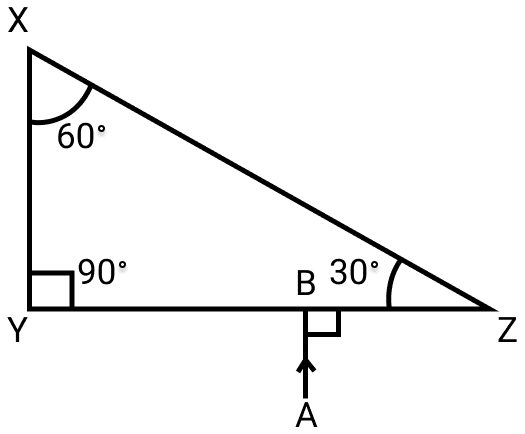The completed diagram is given below: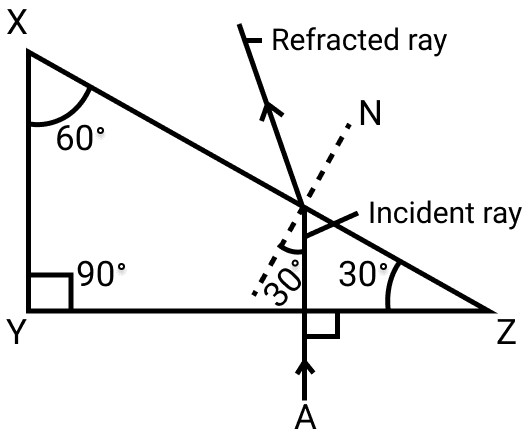#### Question 3(a)

Displacement distance graph of two sound waves A and B, travelling in a medium, are as shown in the diagram below.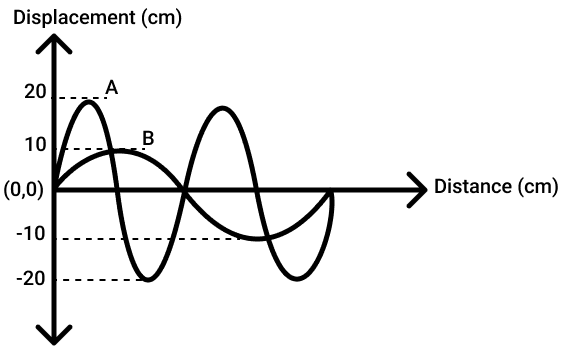Study the two sound waves and compare their:

(i) Amplitudes

(ii) Wavelengths

(i) From figure, amplitude of wave A = 20 cm and

amplitude of wave B = 10 cm.

$\dfrac{\text{Amplitude of wave A}}{\text{Amplitude of wave B}} = \dfrac{20}{10} = 2 : 1$

(ii) Wavelength of A = x and wavelength of B = 2x

$\dfrac{\text{Wavelength of wave A}}{\text{Wavelength of wave B}} = \dfrac{x}{2x} = 1 : 2$

#### Question 3(b)

You have three resistors of values 2 Ω, 3 Ω and 5 Ω. How will you join them so that the total resistance is more than 7 Ω? 

(i) Draw a diagram for the arrangement.

(ii) Calculate the equivalent resistance.

(i) The three resistors are joined in series as shown in the diagram below: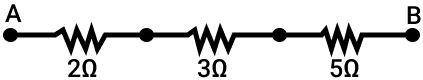(ii) The equivalent resistance R = R1 + R2 + R3

R = 2 + 3 + 5 = 10 Ω

#### Question 3(c)

(i) What do you understand by the term nuclear fusion? 

(ii) Nuclear power plants use the nuclear fission reaction to produce electricity. What is the advantage of producing electricity by fusion reaction?

(i) The combining of two or more light nuclei at very high temperature and pressure, to form a heavy nucleus is called nuclear fusion. In this process, huge amount of energy is released.

(ii) The energy released in nuclear fusion will be more than in nuclear fission for the same mass.

#### Question 3(d)

(i) What do you understand by free vibrations of a body? 

(ii) Why does the amplitude of a vibrating body continuously decrease during damped vibrations?

(i) The periodic vibrations of constant amplitude and frequency of a body in the absence of any external force on it, are called free vibrations.

(ii) The amplitude of a vibrating body continuously decrease during damped vibrations. It is due to loss of energy against the damping forces such as air, friction etc.

#### Question 3(e)

(i) How is the e.m.f. across primary and secondary coils of a transformer related with the number of turns of coil in them? 

(ii) On which type of current do transformers work?

(i) Relation between e.m.f. across primary and secondary coils of a transformer is given below:

$\dfrac{\text{emf across primary coil}}{\text{emf across secondary coil}} = \dfrac{\text{no. of turns in primary coil}}{\text{no. of turns in secondary coil}}$

(ii) Transformers work only with alternating current.

#### Question 4(a)

(i) How can a temperature in degree Celsius be converted into S.I. unit of temperature?

(ii) A liquid X has the maximum specific heat capacity and is used as a coolant in Car radiators. Name the liquid X. 

(i) The temperature in degree Celsius (t) can be converted to S.I. unit Kelvin (T) by adding 273 to it.

T(K) = 273 + (t °C).

(ii) The liquid X is Water.

#### Question 4(b)

A solid metal weighing 150 g melts at it's melting point of 800 °C by providing heat at the rate of 100 W. The time taken for it to completely melt at the same temperature is 4 min. What is the specific latent heat of fusion of the metal? 

Given,

mass m = 150 g = 0.15 kg,

power P = 100 W

time t = 4 min = 4 x 60 = 240 s

energy supplied Q = power x time = 100 x 240 = 24000 J

specific latent heat L = $\dfrac{Q}{m}$ = $\dfrac{24000}{0.15}$ = 1.6 x 10 5 J kg-1

#### Question 4(c)

Identify the following wires used in a household circuit: 

(i) The wire is also called as the phase wire.

(ii) The wire is connected to the top terminal of a three-pin socket.

(i) The wire which is also called the phase wire is the live wire.

(ii) The wire which is connected to the top terminal of a three-pin socket is the earth wire.

#### Question 4(d)

(i) What are isobars?

(ii) Give one example of isobars. 

(i) Isobars are the atoms of same mass number but different atomic number.

(ii) $_{11}^{23}\text{Na}$ and $_{12}^{23}\text{Mg}$ are isobars.

#### Question 4(e)

State any two advantages of electromagnets over permanent magnets. 

The two advantages of electromagnets over permanent magnets are :

1. An electromagnet can be made to produce a strong magnetic field (increasing the number of turns in the coil).
2. The polarity of the electromagnet can be reversed.

## Section II (40 Marks)

#### Question 5(a)

(i) Derive a relationship between S.I. and C.G.S. unit of work. 

(ii) A force acts on a body and displaces it by a distance S in a direction at an angle θ with the direction of force. What should be the value of θ to get the maximum positive work?

S.I. unit of work is joule and C.G.S. unit of work is erg.

Since, work = Force x displacement

1 joule = 1 newton x 1 metre

= 105 dyne x 100 cm

= 107 dyne x cm

= 107 erg

1 joule = 107 erg

(ii) Work W = F.S. cos θ

For maximum work, cos θ = 1 or θ = 0°

#### Question 5(b)

A half metre rod is pivoted at the centre with two weights of 20 gf and 12 gf suspended at a perpendicular distance of 6 cm and 10 cm from the pivot respectively as shown below.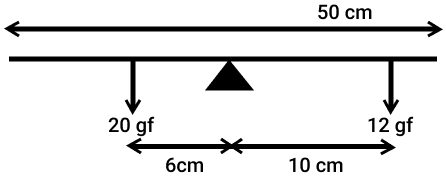(i) Which of the two forces acting on the rigid rod causes clockwise moment?

(ii) Is the rod in equilibrium?

(iii) The direction of 20 gf force is reversed. What is the magnitude of the resultant moment of the forces on the rod?

(i) The force 12 gf rotates the rod clockwise.

(ii) Since, the clockwise moment (= 20 gf x 6 cm = 120 gf cm) is equal to the anticlockwise moment (= 12 gf x 10 cm = 120 gf cm), hence the rod is in equilibrium.

(iii) On reversing the direction of force, 20 gf will also produce clockwise moment, total clockwise moment will then be (20 gf x 6 cm + 12 gf x 10 cm) = 240 gf cm

#### Question 5(c)

(i) Draw a diagram to show a block and tackle pulley system having a velocity ratio of 3 marking the direction of load (L), effort (E) and tension (T). 

(ii) The pulley system drawn lifts a load of 150 N when an effort of 60 N is applied. Find it's mechanical advantage.

(iii) Is the above pulley system an ideal machine or not?

(i) The block and tackle pulley system with velocity ratio 3 is shown below in which the direction of load (L), effort (E) and tension (T) has been marked: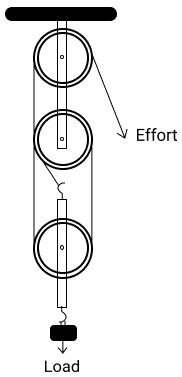(ii) Given,

L = 150 N,

E = 60 N

Mechanical advantage M.A. = $\dfrac{𝐿}{E}$ = $\dfrac{150}{60}$ = 2.5

(iii) This pulley system is not ideal.

#### Question 6(a)

A ray of light XY passes through a right angled isosceles prism as shown below.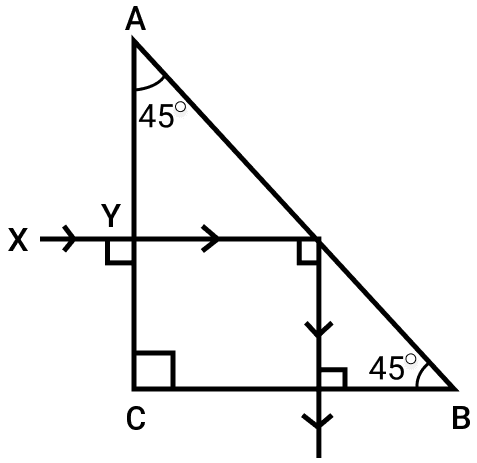(i) What is the angle through which the incident ray deviates and emerges out of the prism?

(ii) Name the instrument where this action of prism is put into use.

(iii) Which prism surface will behave as a mirror?

(i) Angle of deviation = the angle which the emergent ray makes with the incident ray XY = 90°.

(ii) Periscope

(iii) The surface AB of the prism behaves as the mirror.

#### Question 6(b)

An object AB is placed between O and F1 on the principal axis of a converging lens as shown in the diagram.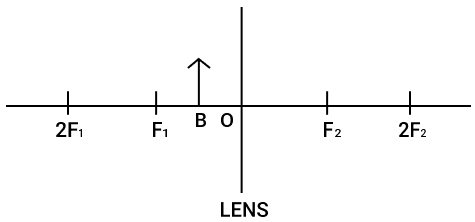Copy the diagram and by using three standard rays starting from point A, obtain an image of the object AB.

The completed diagram is given below :#### Question 6(c)

An object is placed at a distance of 12 cm from a convex lens of focal length 8 cm. 

Find:

(i) the position of the image

(ii) nature of the image

(i) Given,

u = 12 cm (negative),

f = 8 cm (positive)

From lens formula

$\dfrac{1}{v} - \dfrac{1}{u} = \dfrac{1}{f}$

Substituting the values we get,

$\dfrac{1}{v} - \dfrac{1}{-12} = \dfrac{1}{8} \\[0.5em] \Rightarrow \dfrac{1}{v} + \dfrac{1}{12} = \dfrac{1}{8} \\[0.5em] \Rightarrow \dfrac{1}{v} = \dfrac{1}{8} - \dfrac{1}{12} \\[0.5em] \Rightarrow \dfrac{1}{v} = \dfrac{3 - 2}{24} \\[0.5em] \Rightarrow \dfrac{1}{v} = \dfrac{1}{24} \\[0.5em] \Rightarrow v = 24 \text{ cm}\\[0.5em]$

The image will be formed at a distance 24 cm behind the lens.

(iii) The image will be real, inverted and magnified.

#### Question 7(a)

Draw the diagram of a right angled isosceles prism which is used to make an inverted image erect. 

Below is the diagram of a right angled isosceles prism which is used to make an inverted image erect: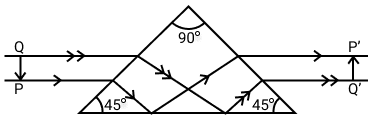#### Question 7(b)

The diagram below shows a wire stretched over a sonometer. Stems of two vibrating tuning forks A and B are touched to the wooden box of the sonometer. It is observed that the paper rider (a small piece of paper folded at the centre) present on the wire flies off when the stem of vibrating tuning fork B is touched to the wooden box but the paper just vibrates when the stem of vibrating tuning fork A is touched to the wooden box.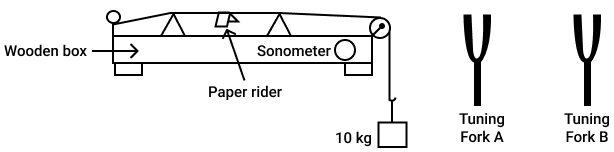(i) Name the phenomenon when the paper rider just vibrates.

(ii) Name the phenomenon when the paper rider flies off.

(iii) Why does the paper rider fly off when the stem of tuning fork B is touched to the box?

(i) The tuning fork A produces forced vibrations in the wire due to which the rider vibrates.

(ii) The tuning fork B produces resonant vibrations in the wire due to which the rider flies off.

(iii) The frequency of tuning fork B is equal to the natural frequency of wire, so resonance occurs and the wire vibrates with a large amplitude and rider flies off.

#### Question 7(c)

A person is standing at the sea shore. An observer on the ship which is anchored in between a vertical cliff and the person on the shore fires a gun. The person on the shore hears two sounds, 2 seconds and 3 seconds after seeing the smoke of the fired gun. If the speed of sound in the air is 320 ms-1 then calculate: 

(i) the distance between the observer on the ship and the person on the shore.

(ii) the distance between the cliff and the observer on the ship.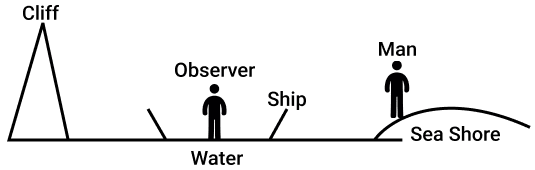Let d1 be the distance between the observer on ship and person on shore. The first sound heard by the person at shore after t1 = 2 s will be the direct sound of fire.

From relation v = $\dfrac{d}{t}$,

320 = $\dfrac{d_1}{2}$ or d1 = 640 m.

(ii) The second sound heard by the person at shore will be the echo heard after reflection from the cliff. Since, sound takes 2 s to reach from the observer to the person, so the time taken by the echo of fire to reach the observer will be t2 = 3 - 2 = 1 s.

Let d2 be the distance between the observer and the cliff. Then $\dfrac{2d_2}{t_2}$ = v

Or d2 = $\dfrac{vt_2}{2}$ = $\dfrac{320 \times 1}{2}$ = 160 m.

#### Question 8(a)

(i) A fuse is rated 8A. Can it be used with an electrical appliance rated 5 KW, 200 V? Give a reason. 

(ii) Name two safety devices which are connected to the live wire of a household electric circuit.

Given,

P = 5 KW = 5000 W,

V = 200 V

Current drawn i = $\dfrac{P}{V}$ = $\dfrac{5000 W}{200 V}$ = 25 A

Since, the appliance when in use will draw 25 A current, the fuse rated 8 A cannot be used because it will blow off as the current will exceed 8 A and the circuit wil break.

(ii) Safety devices which are connected to the live wire of a household electric circuit are switch and fuse.

#### Question 8(b)

(i) Find the equivalent resistance between A and B.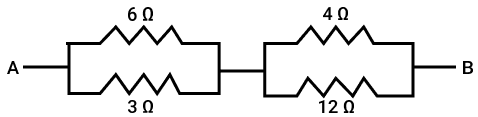(ii) State whether the resistivity of a wire changes with the change in the thickness of the wire.

(i) In figure, a parallel combination of resistors 6 Ω and 3 Ω are connected in series with another parallel combination of resistors 4 Ω and 12 Ω between the terminals A and B.

In the first part, let the equivalent resistance of parallel combination of resistors 3 Ω and 6 Ω be R1.

$\dfrac{1}{R_1} = \dfrac{1}{3} + \dfrac{1}{6} \\[0.5em] \Rightarrow \dfrac{1}{R_1} = \dfrac{2 + 1}{6} \\[0.5em] \Rightarrow \dfrac{1}{R_1} = \dfrac{3}{6} \\[0.5em] \Rightarrow \dfrac{1}{R_1} = \dfrac{1}{2} \\[0.5em] \Rightarrow R_1 = 2 Ω$

In the second part, 4 Ω and 12 Ω are in parallel connection. If the equivalent resistance is R2 then

$\dfrac{1}{R_2} = \dfrac{1}{4} + \dfrac{1}{12} \\[0.5em] \Rightarrow \dfrac{1}{R_2} = \dfrac{3 + 1}{12} \\[0.5em] \Rightarrow \dfrac{1}{R_2} = \dfrac{4}{12} \\[0.5em] \Rightarrow \dfrac{1}{R_2} = \dfrac{1}{3} \\[0.5em] \Rightarrow R_2 = 3 Ω$

In the third part R1 and R2 are in series. Therefore we get,

2 + 3 = 5 Ω

Hence, equivalent resistance between A and B = 5 Ω.

(ii) The resistivity of wire does not change with the change in the thickness of the wire.

#### Question 8(c)

An electric iron is rated 220V, 2 kW. 

(i) If the iron is used for 3h daily, find the cost of running it for one week if it costs ₹4.25 per kWh.

(ii) Why is the fuse absolutely necessary in a power circuit?

Given,

power P = 2 kW,

time t = 2h,

number of days = 7 and cost = ₹4.25 per kWh

Electrical energy consumed = Power x total time
= 2 kW x (2 x 7) h
= 28 kWh

Cost = 28 x 4.25 = ₹119

∴ Cost of running the iron for a week is ₹119

(ii) The appliances connected to the power circuit like air conditioner, geyser, washing machine, etc. consume heavy current. The fuse is absolutely necessary in a power circuit because if somehow the circuit is overloaded (i.e., the total power of appliances connected in the circuit exceeds the power limit of the circuit) an excessive current will flow in the circuit and the line wire or appliances may get damaged. Moreover, due to the excessive heat produced by such high current, the wires may get overheated causing a fire. The use of fuse in power circuit will safe guard against any such mishap.

#### Question 9(a)

(i) Heat supplied to a solid change it into liquid. What is this change in phase called? 

(ii) During the phase change does the average kinetic energy of the molecules of the substance increase?

(iii) What is the energy absorbed during the phase change called?

(i) The change of phase from solid to liquid on heating is called melting.

(ii) No, the average kinetic energy of the molecules does not change during the change of phase.

(iii) The energy absorbed during the phase change is called latent heat.

#### Question 9(b)

(i) State two differences between "Heat Capacity" and "Specific Heat Capacity". 

(ii) Give a mathematical relation between Heat Capacity and Specific Heat Capacity.

(i) Difference between "Heat Capacity" and "Specific Heat Capacity" are as follows :

Heat CapacitySpecific Heat Capacity
It is the amount of heat energy required to raise the temperature of entire body by 1 °C.It is the amount of heat energy required to raise the temperature of unit mass of the body by 1 °C.
The S.I. unit is JK-1S.I. unit is J kg-1K-1.

(ii) Heat Capacity = Mass x Specific Heat Capacity

#### Question 9(c)

The temperature of 170 g of water at 50°C is lowered to 5°C by adding a certain amount of ice to it. Find the mass of ice added.

Given: Specific heat capacity of water = 4200 J kg-1 ⁰C-1 and Specific latent heat of ice = 336000 J kg-1 

Given,

mass of water m = 170 g = 0.17 kg

initial temperature = 50°C

final temperature = 5°C

fall in temperature = (50 - 5) = 45°C = 45 K

Heat lost by water = m x c x Δt
= 0.17 x 4200 x 45
= 3.213 x 104

If m' kg ice is added, heat gained by it to melt to 0°C = m'L
= m' x 3.36 x 105

Heat gained by it to raise temperature by 5°C = m' x C x Δt
= m' x 4200 x 5
= m' x 2.1 x 104 J

Total heat gained by ice = (m' x 3.36 x 105) + (m' x 2.1 x 104)

= m' x 3.57 x 105 J

By the principle of method of mixtures:
heat lost by water = heat gained by ice

$3.213 \times 10^4 = \text{m}' \times 3.57 \times 10^5 \\[0.5em] \text{m}' = \dfrac{3.213 \times 10^4}{3.57 \times 10^5} \\[0.5em] \text{m}' = 0.09 \text{ kg} = 90 \text{ g}$

Hence, mass of ice added = 90 g

#### Question 10(a)

The diagram shows a coil wound around a U shape soft iron bar AB.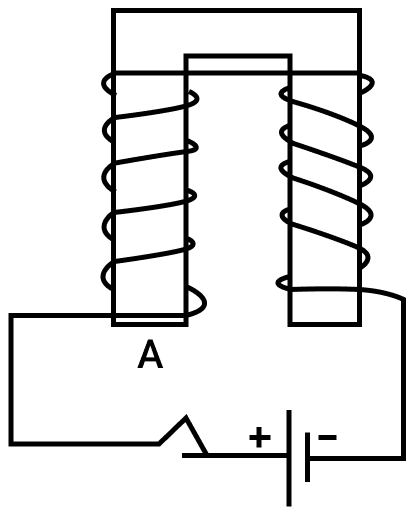(i) What is the polarity induced at the ends A and B when the switch is pressed?

(ii) Suggest one way to strengthen the magnetic field in the electromagnet.

(iii) What will be the polarities at A & B if the direction of current is reversed in the circuit?

(i) The polarity induced at the end A is North and at the end B is South.

(ii) To strengthen the magnetic field in the electromagnet, the current flowing in it is increased.

(iii) On reversing the direction of current, the end A will be south pole and the end B will be the north pole.

#### Question 10(b)

The ore of Uranium found in nature contains $_{92}^{238}\text{U}$ and $_{92}^{235}\text{U}$. Although both the isotopes are fissionable, it is found out experimentally that one of the two isotopes is more easily fissionable. 

(i) Name the isotope of Uranium which is easily fissionable.

(iii) Write a nuclear reaction when Uranium 238 emits an alpha particle to form a Thorium (Th) nucleus.

(i) $_{92}^{235}\text{U}$ is easily fissionable.

(ii) The reason is that the fission of $_{92}^{238}\text{U}$ nucleus is possible only by the fast neutrons, while the fission of $_{92}^{235}\text{U}$ nucleus can be done by both slow as well as fast neutrons.

(iii) $_{92}^{238}\text{U}$$_{90}^{234}\text{Th}$ + $_{2}^{4}\text{He}$

#### Question 10(c)

Radiations given out from a source when subjected to an electric field in a direction perpendicular to their path are shown in the diagram. The arrows show the path of the radiation A, B and C. Answer the following question in terms of A, B and C.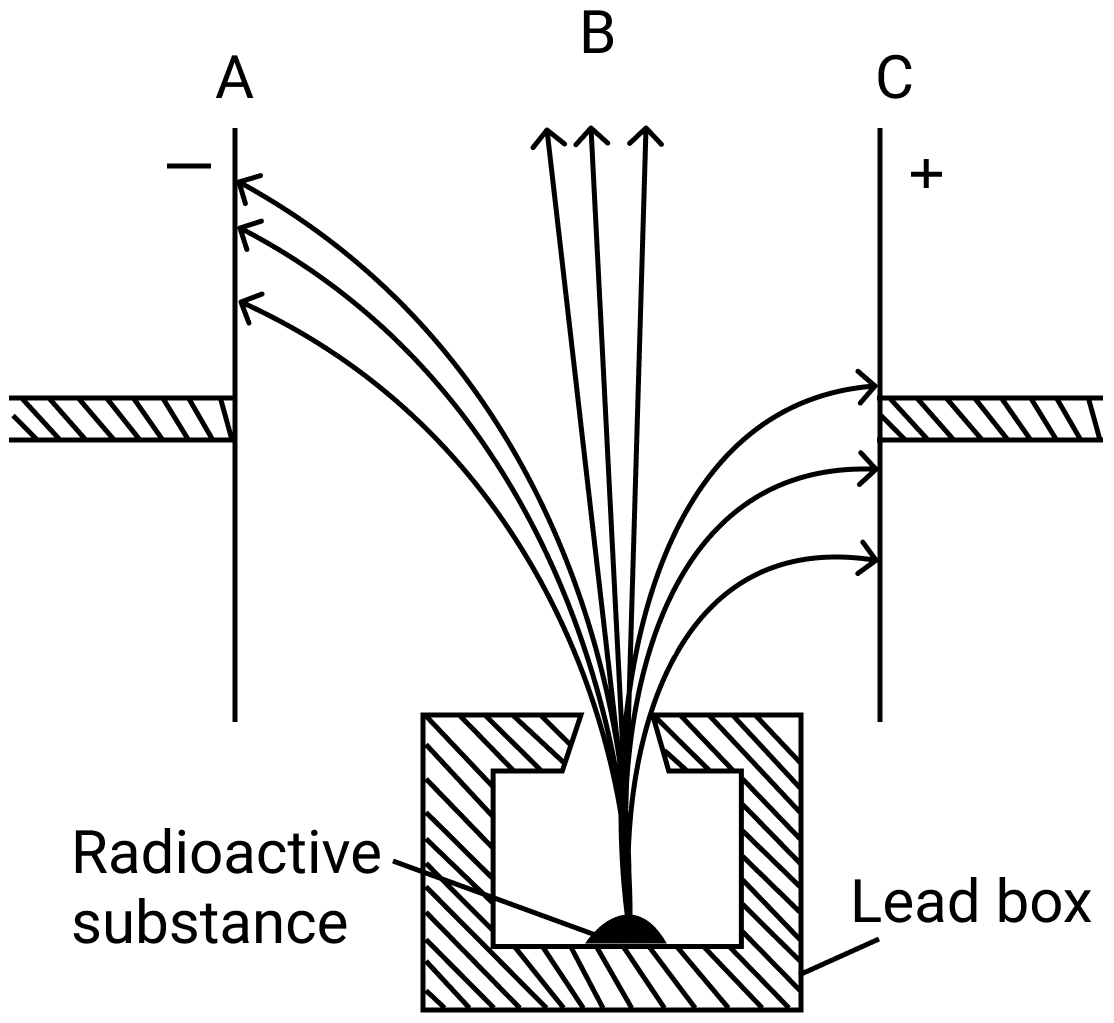(i) Name the radiation B which is unaffected by the electrostatic field.

(ii) Why does the radiation C deflect more than A?

(iii) Which among the three causes the least biological damage externally.

(iv) Name the radiation which is used in carbon dating.